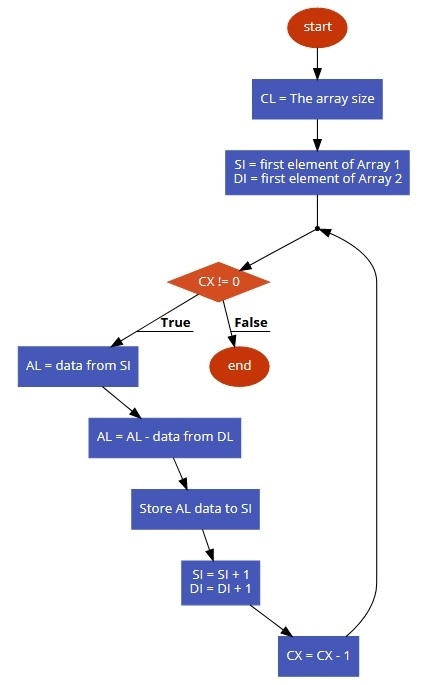# 8086 program to determine subtraction of corresponding elements of two arrays

In this program we will see how to subtract the contents of two different arrays.

## Problem Statement

Write 8086 Assembly language program to subtract the contents to corresponding elements which are stored in two different arrays

## Discussion

In this example there are two different arrays. The arrays are stored at location 501 onwards and 601 onwards. The size of these two arrays are stored at offset location 500. We are taking the array size to initialize the counter, then by using loops we are subtracting the elements one by one

50004
50109
50203
50308
50406
60104
60201
60302
60403

## Flow Diagram## Program

    MOV SI, 500     ;Point Source index to 500
MOV CL, [SI]    ;Load the array size into CL
MOV CH, 00 ;Clear Upper half of CX
INC SI   ;Increase SI register to point next location
MOV DI, 601     ;Destination register points to 601
L1: MOV AL, [SI]    ;Load A with the data stored at SI
SUB AL, [DI]    ;Subtract DI element from AL
MOV [SI], AL    ;Store AL to SI address
INC SI   ;SI Point to next location
INC DI   ;DI Point to next location
LOOP L1     ;Jump to L1 until the counter becomes 0
HLT ;Terminate the program